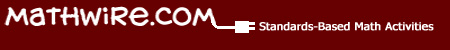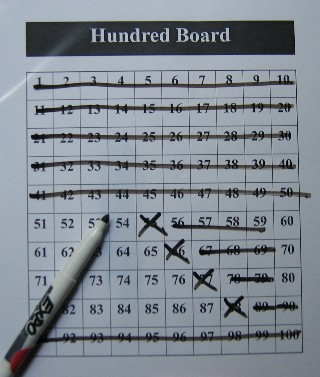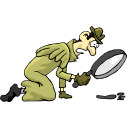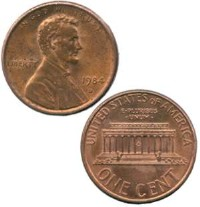Problem Solving: 100 Board Logic ProblemsHundred Board Logic

Strategy: Students use a hundred board to eliminate numbers after reading each clue.   Students must apply their knowledge of even-odd, multiples and place value to successfully eliminate numbers until the solution is revealed.

Materials:

• Copy ofHundred Board Logic packet for teacher reference
• Overhead of Cooperative Learning Roles (found in teacher packet)
• Copies of problems for each cooperative learning group (found in teacher packet)
•Hundred Board for each group.   Place each chart in a clear sleeve protector so that students can use dry erase markers to cross out numbers after reading each clue.
• dry-erase markers and erasers for each group
• Hundred Board transparency or dry-erase Hundred Board Chart for instructional use
• Copies of the blank hundred board logic problem template for students to write their own problems

Introducing the Lesson:

`
• Identify cooperative learning groups of 3-4 students and appoint roles or have students pick numbers 1-4 and assign roles using those numbers (e.g. all 1s are the recorder, etc.)
• Use the transparency to discuss the cooperative learning group roles and the responsiblity of each member in the problem solving process
• Pick one role from each group to form a class "group" to model the first problem which students will solve as a whole-class.
• Only the reader has a copy of the problem which he/she reads aloud to the class, pausing after each clue so that the group can decide which numbers to cross out for that clue.
• The recorder crosses out the numbers on the class hundred board and the checkers make sure that all work is correct.
• When the group is finished and has arrived at one answer, model having the reader reread the problem clue by clue. Students should signal thumbs up/thumbs down that their answer satisfies that clue. Discuss with students how taking the time to check their work clue by clue means they can't get a wrong answer because if their answer is wrong, one of the clues won't work.
• The leader then brings the completed hundred board with the answer circled to the teacher for checking. If correct, give the group the next problem. If the answer is incorrect, circle the clue that does not work and ask the group to do the problem again.
• Review cooperative group roles, if necessary.

Cooperative Learning Group Problem Solving:

• Distribute materials to all groups.
• Give each reader a copy of the next problem and instruct groups to begin working.
• Circulate around the room as students work to be sure that all students are actively involved and observing assigned roles.
• As a group supplies the correct answer and label, give them the next problem.

Writing Hundred Board Logic Problems:

• When a group finishes all problems, they should be given blank templates so that they can begin to write their own problems modeled on the problems done in class. They begin with a short blurb, pick an answer and then write clues one at a time until their answer is the only number left.
• Each student should exchange his/her problem with a partner for editing. Each student works out their partner's problem to see if he/she agrees with the answer or if there are inconsistencies that need to be resolved.
• Have students publish the problems with their names, grade and school year so that you accumulate a bank of these problems for future students.Guess The Number

Give students more practice in using the hundred board to solve number clue problems. Once again, students solve the problem clue by clue. With this set, however, students must use multiplication, money, and measurement facts to correctly solve the problem and guess the number.

• DownloadGuess the Number teacher resource packet.Sample Problem:Guess the Number-1

• The number is greater than the number of pennies in a quarter.
• The number is less than the number of pennies in five dimes.
• The number is an odd number.
• If you count by 5s, you say the number.
• The sum of the digits is 8.
• What is the number?

Suggested Uses:

• Do Now! or warm-up activity
• Math Center Activity
• Problem solving activity on game days
• Writing original Guess The Number problems for a class collection